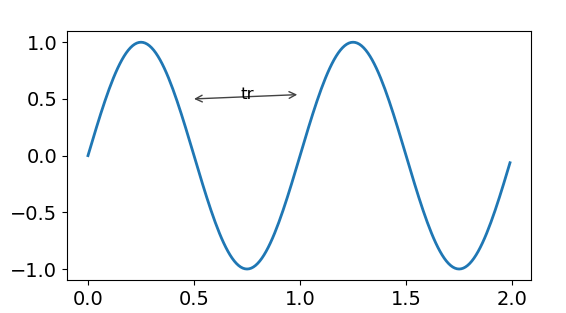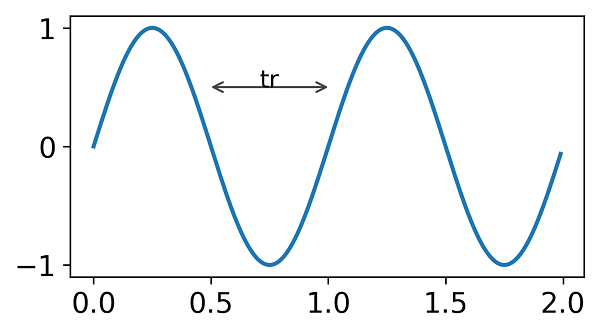# Unable to draw horizontal arrow using annotation #9591

Closed
opened this issue Oct 27, 2017 · 3 comments

Projects
None yet

### Bug report

Unable to draw straight horizontal arrow using annotation

Code for reproduction

``````
from pylab import figure, show, np

t = np.arange(0.0, 2.0, 0.01)
s1 = np.sin(2*np.pi*t)
fig = figure()
xstmark=0.5
xenmark=1.0
ystmark=0.5
an1=ax1.annotate(s=' ',xy=(xstmark, ystmark), xycoords='data',
xytext=(xenmark, ystmark),textcoords='data',
arrowprops=dict(arrowstyle="<->"))
ax1.annotate(s='tr',xy=(((xstmark+xenmark)/2), ystmark), xycoords='data',fontsize=12.0,textcoords='data',ha='center')
ax1.plot(t, s1)

show()
``````

Matplotlib 2.1.0 outcomeExpected outcome (Matplotlib 2.0.2 outcome)• Operating system: Windows 7 64 bit
• Matplotlib version: 2.1.0
• Matplotlib backend: qt4agg
• Python version: 2.7.10
• Jupyter version (if applicable): NA
• Other libraries: NA
• Installed using whl files
Contributor

### anntzer commented Oct 27, 2017 • edited

 Using some text (e.g. `'foo'`) for the annotation shows the origin of the "problem":the arrow is pointing towards the center of the text, not the baseline (which seems very reasonable behavior). You are using a space (`' '`) as text, and we consider the y-center of thet space as the same as the y-center of a letter (more precisely, the y-center of `'lp'`) (see Text._get_layout: "full vertical extent of the font, including ascenders and descenders"). If using an empty string instead (`''`), then we consider the y-center to be at the baseline (which in fact seems a bit inconsistent; I'd think empty strings should just be treated the same -- in other words, right now you can have the workaround of using `''` instead of `' '` but I'm suggesting to break that case too). Not special casing strings with just spaces was done in #6005. Of course, the fact that `annotate` is the "recommended" API to get a FancyArrowPatch rather than a FancyArrow (see bottom of https://matplotlib.org/api/_as_gen/matplotlib.axes.Axes.arrow.html) is not a great API, nor is the fact that FancyArrow and FancyArrowPatch are actually completely different things :/
Author

### inxp commented Oct 27, 2017

 Thanks for replying... It makes sense. I'll use the workaround for now :)
Author

### inxp commented Oct 27, 2017 • edited

 or I can add va='center' while drawing arrow. This works with space and empty string. ``````an1=ax1.annotate(s=' ',xy=(xstmark, ystmark), xycoords='data', xytext=(xenmark, ystmark),textcoords='data', arrowprops=dict(arrowstyle="<->"),va='center') ``````